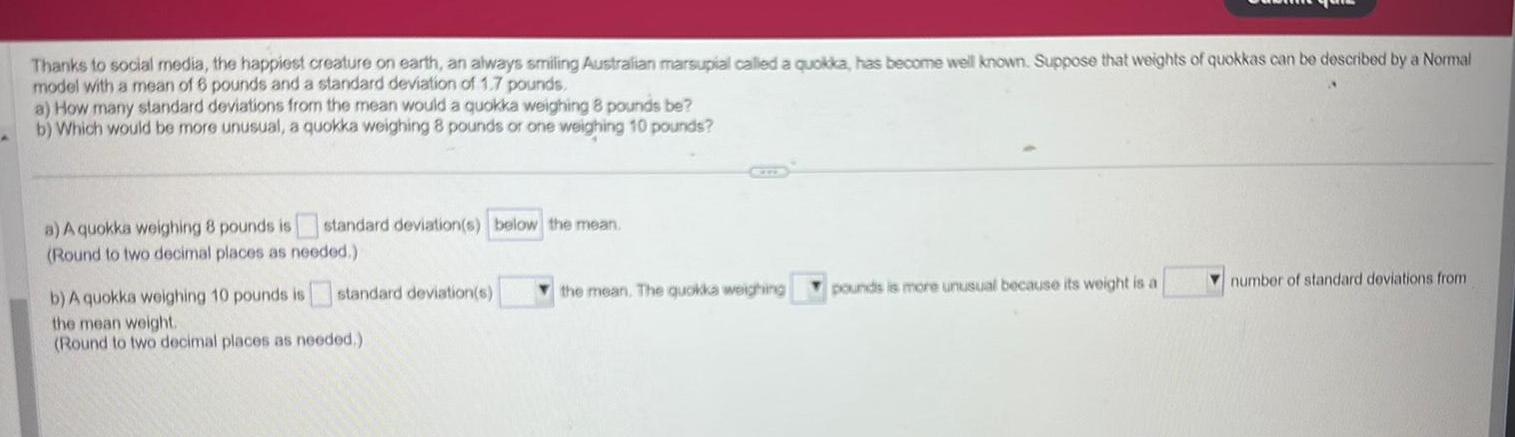Question:

# Thanks to social media the happiest creature on earth an

Last updated: 9/18/2023Thanks to social media the happiest creature on earth an always smiling Australian marsupial called a quokka has become well known Suppose that weights of quokkas can be described by a Normal model with a mean of 6 pounds and a standard deviation of 1 7 pounds a How many standard deviations from the mean would a quokka weighing 8 pounds be b Which would be more unusual a quokka weighing 8 pounds or one weighing 10 pounds a A quokka weighing 8 pounds is standard deviation s below the mean Round to two decimal places as needed b A quokka weighing 10 pounds is standard deviation s the mean weight Round to two decimal places as needed the mean The quokka weighing pounds is more unusual because its weight is a number of standard deviations from